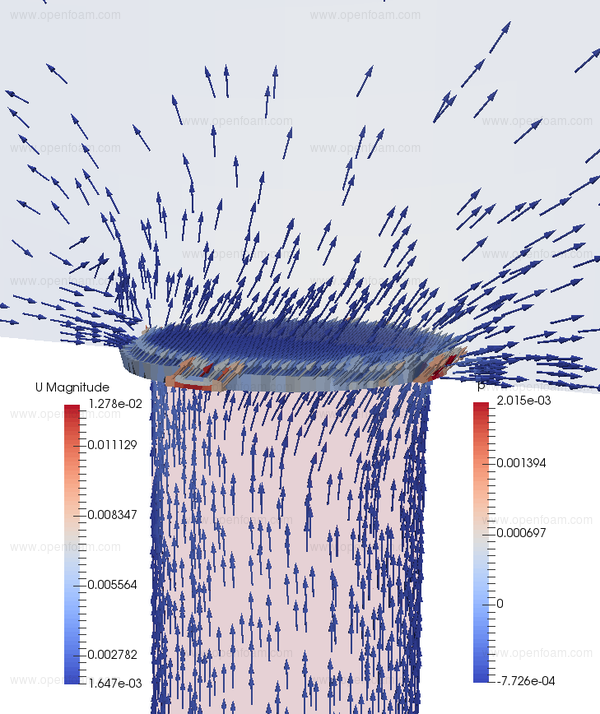This source deflects the flow direction by imposing a variable pressure drop. This is particularly useful for cases that include louvres, e.g. HVAC registers, whereby to resolve the louvres using the mesh can lead to excessive cell counts and excessively restrict the calculation time step.

## Properties🔗

The pressure drop across the cellZone is evaluated according to the options:

• constant: user-specified $$\Delta p$$ [Pa]
• volumetricFlowRateTable: $$\Delta p$$ is read as a table from file
• DarcyForchheimer: $$\Delta p = (D + 0.5 \rho |U| I ) |U| L$$ [Pa] where D, I and L are user inputs.

• the cellZone should describe a set of cells with a 1-cell thickness in the flow direction
• the source requires the set of faces upstream of the cellZone to be specified as a faceZone

A sample result is shown below, where the effect of flow deflection across the cellZone is clearly evident.## Usage🔗

The option is specified using:

registerOrientation
{
active              true;
selectionMode       cellZone;
cellZone            c1Zone;
fieldNames          (U);
flowDir             (1 2 0);    // new flow direction in the general coordinate system
relaxationFactor    0.3;        // relax the application of the source term
faceZone            f1Zone;     // faceZone upstream the cellZone

// Pressure drop model $Pa$
model               DarcyForchheimer;   // volumetricFlowRateTable | constant

// DarcyForchheimer
// deltaP = (D*mu + 0.5*rho*magUn)*magUn*length
D                   5e7;
I                   0;
length              0.01;  // Virtual thickness of the porosity corresponding to the D and I values

// constant pressure drop model
// pressureDrop    8;

// volumetricFlowRateTable pressure drop model
// outOfBounds     clamp;
// file            "volFlowRateTable";
}


Tutorials

Source code